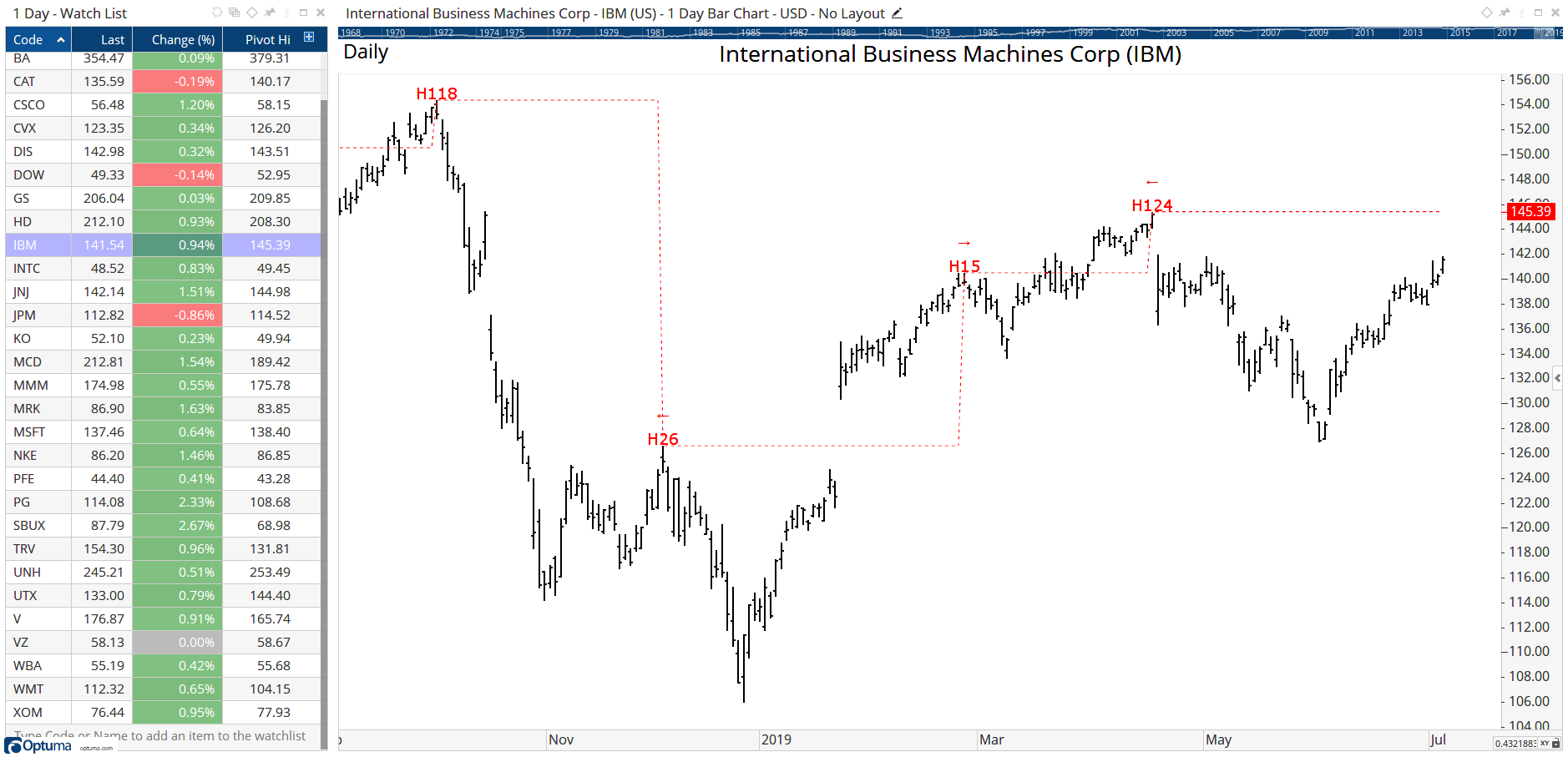# Pivot() Function

Author: Optuma Team Last updated: Jul 17, 2021 01:35

The Pivot function uses the same functionality as the Pivot Labels tool to identify turning points in either a price chart or an indicator.

To calculate the value of the last confirmed 15 bar pivot high, and show in a watchlist column or Show Plot:

``````//Define the pivot criteria
V1 = PIVOT(MIN=15, TYPE=High, IGNOREUNCONFIRMED=True);
//Get the high price when the last V1 pivot occurred
VALUEWHEN(HIGH(), V1)
``````To scan for when the closing price crosses below a confirmed 10 bar pivot low:

``````//Define the pivot criteria
V1=PIVOT(MIN=10, TYPE=Low, IGNOREUNCONFIRMED=True);
//Get the low price when the last V1 pivot occurred
V2=VALUEWHEN(LOW(), V1);
CLOSE() CrossesBelow V2
``````

To highlight on a the price chart when the RSI(14) has a 20 bar pivot low using a Show Bar (note pivot lows have a negative number, so need to be below 0 to be true):

``````PIVOT(RSI(BARS=14),MIN=15, TYPE=Low)
``````

### Blog Posts

See these blog posts on using the Pivot function to find breakouts and divergences:

An Easy Way to Find Breakouts

Identifying Divergences